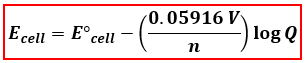# Problem: A concentration cell consists of two Sn/Sn2+ half-cells. The cell has a potential of 0.15V at 25°C. What is the ratio of the Sn2+ concentrations in the two half-cells?

79% (10 ratings)
###### FREE Expert Solution

We are asked to find the ratio of the Sn2+ concentrations in the two Sn/Sn2+ half-cells. We will use the Nernst Equation to calculate for the ratio. In the Nernst Equation, we will calculate for Q where Q is equal to [products]/[reactants].

The Nernst Equation at 25°C:Ecell = cell potential under non-standard conditions
cell = standard cell potential
n = number of e- transferred
Q= reaction quotient = [products]/[reactants]

In the Nernst Equation, the E°cell is needed but only Ecell was given. We have to determine the E°cell first and as well as the anode (oxidation) and cathode (reduction) in the concentration cell and the number of electrons transferred (n).

79% (10 ratings)###### Problem Details

A concentration cell consists of two Sn/Sn2+ half-cells. The cell has a potential of 0.15V at 25°C. What is the ratio of the Sn2+ concentrations in the two half-cells?

What scientific concept do you need to know in order to solve this problem?

Our tutors have indicated that to solve this problem you will need to apply the The Nernst Equation concept. You can view video lessons to learn The Nernst Equation. Or if you need more The Nernst Equation practice, you can also practice The Nernst Equation practice problems.

What is the difficulty of this problem?

Our tutors rated the difficulty ofA concentration cell consists of two Sn/Sn2+ half-cells. The...as medium difficulty.

How long does this problem take to solve?

Our expert Chemistry tutor, Sabrina took 5 minutes and 28 seconds to solve this problem. You can follow their steps in the video explanation above.

What professor is this problem relevant for?

Based on our data, we think this problem is relevant for Professor Tang's class at USF.